Publication date: 05/24/2021

##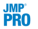Association Analysis Performance Measures

This section defines the performance measures used in the Association Analysis platform. Denote an association rule with condition item set X and consequent item set Y by X Y. Hahsler (2015) contains a collection of performance measures used in association analysis, including support, confidence, and lift.

###Support

Support is the proportion of transactions in which an item set occurs. Support can also be viewed as the probability that a transaction contains an item set.

The support S of a condition item set X is defined as follows: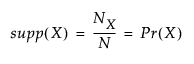where:

NX is the number of transactions that contain the item set X

N is the total number of transactions.

Support for an association rule is defined as follows: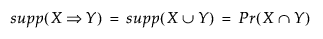In this case, support is equivalent to the probability that a transaction contains both item sets X and Y.

For both the item set and association rule cases, support ranges from 0% to 100%.

###Confidence

Confidence is the proportion of transactions that contain the consequent item set, given that the transaction contains the condition item set. Confidence can also be viewed as the conditional probability that a transaction contains the consequent item set, given that the transaction contains the condition item set.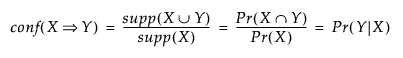Confidence ranges from 0% to 100%. An association rule with a confidence of 0% has a consequent item set that does not appear in any transaction with the condition item set. A confidence of 100% indicates that every transaction that contains the condition item set also contains the consequent item set.

Note: Confidence in association analysis is not related to the concept of confidence intervals.

###Lift

Lift measures dependency between X and Y.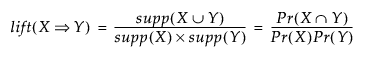The numerator for lift is the proportion of transactions where X and Y occur jointly. The denominator is an estimate of the expected joint occurrence of X and Y, assuming that they occur independently.

Lift ranges from 0 to . A lift value of 1 indicates that X and Y jointly occur in transactions with the frequency that would be expected by chance alone. Increasing lift values suggest that Y occurs more often than expected when X is present.

Note: The lift for association rule X Y is equal to the lift for association rule Y X.